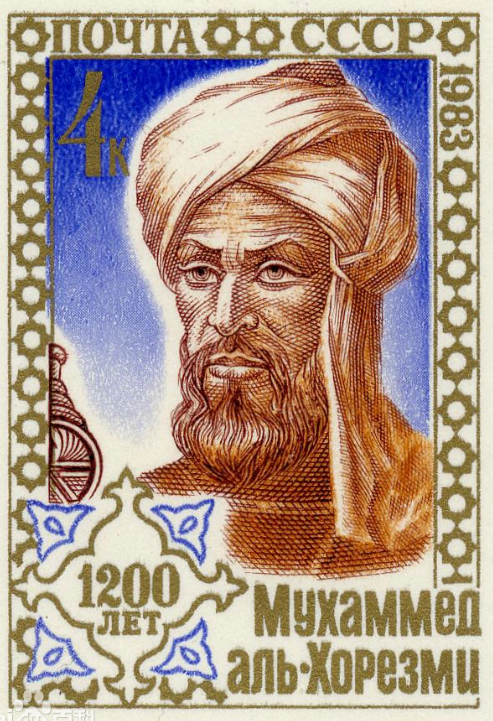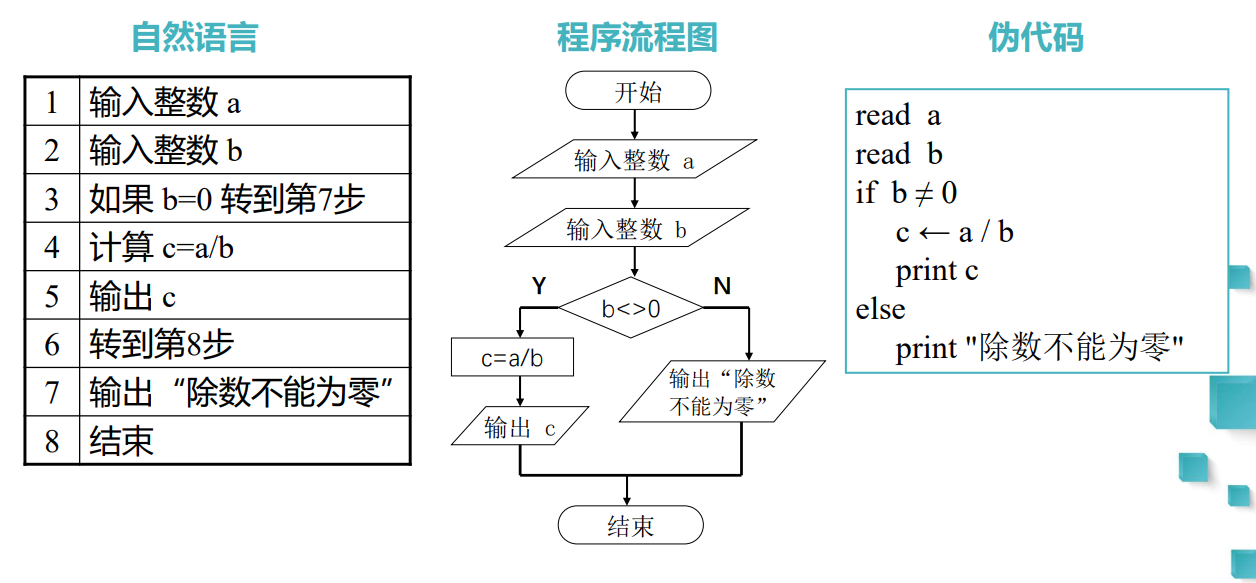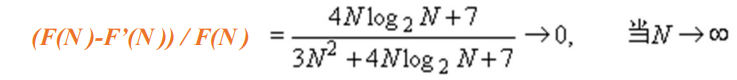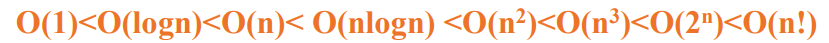> 文档中心 > 『算法导论』什么是算法？什么是程序？

# 『算法导论』什么是算法？什么是程序？

👨‍🎓作者简介：一位喜欢写作，计科专业大二菜鸟

🏡个人主页：starry陆离

🕒首发日期：2022年8月2日星期二

🌌上期文章：『首期文章』

📚订阅专栏：『算法分析与设计』### 『算法导论』什么是算法？什么是程序？

• 1.什么是算法
• 2.“算法”的来源
• 3.什么是程序
• 4.三种常用的描述算法的形式
• 5.算法的好与坏
• 6.算法复杂性的渐近性态
• 7.时间复杂性渐进表示法
• 8.常见的算法复杂度的大O阶

# 1.什么是算法

• 输入：有零个或多个输入

• 输出：至少有一个输出

• 确定性：组成算法的每条指令清晰、无歧义

• 有限性：算法中每条指令的执行次数有限，执行每条指令的时间也有限

# 2.“算法”的来源• 程序不一定满足有限性

# 4.三种常用的描述算法的形式

• 优点：通俗易懂
• 缺点：但缺乏直观性和简洁性，且易产生歧义。
• 举例： “南京市长江大桥”、“这个人连我都不认识”、“我曾经喜欢一个人，我现 在喜欢一个人”……

• 优点：描述算法形象、直观，容易理解，
• 绘制过程比较费时费力，难 以修改。

• 优点：简单易懂，修改容易，易于转化为程序语言代码
• 缺点：伪代码格式难以规范# 6.算法复杂性的渐近性态F’(N)的一个答案是3N2，

# 7.时间复杂性渐进表示法

O的定义：如果存在正的常数C和自然数N0，使得当N >= N0时有f(N) <= Cg(N)，则称函数f(N)当N充分大时上有界，且g(N)是它的一个上界，记为 `f(N)=O(g(N))`，即f(N)的阶不高于g(N)的阶

• 对所有N >=1时，3N <=4N，故3N=O(N)

• 对所有N>=1时，N+1024 <=1025N，故N+1024=O(N)

• 对所有N >=10时，2N2+11N-10 <=3N2，故2N2+11N-10=O(N2 )

• 对所有N >=1时，N2 <=N3，故N2=O(N3 )

A. f(n)=O(n) • B. f(n)=O(1/n) • C. f(n)=O(n2 ) • D. f(n)=O(n3 )

Ω的定义：如果存在正的常数C和自然数N0，使得当N >= N0时有f(N) >= Cg(N)，则称函数f(N)当N充分大时下有界，且g(N)是它的一个下界，记为 `f(N)=Ω(g(N))`，即f(N)的阶不低于g(N)的阶。

• 1024n+1024 = Ω(n)
• n2+n+1 = Ω(n)
• 2n3+nlogn+1 = Ω(n2 )

• (1) 5n2 + 10n =O(n2)

• (2) n + nlogn2=O(nlogn)

• (3) n2 /100 + 3n =(O(n2))

• (4) 20 log3n =O(n)

• (5) 1/n2 + 1 = O(1)

• (6) logn5 + n1/2 = O(logn)

# 8.常见的算法复杂度的大O阶

O(1): 表示算法的运行时间为常量时间

O(logn): 二分查找等算法

O(n): 线性算法，例如线性查找

O(nlogn): 快速排序、归并排序等算法

O(n2 ): 对数组进行排序的简单算法，例如冒泡排序

O(n3 ): 做两个n阶矩阵的乘法运算

O(2n ): 求具有n个元素集合的所有子集的算法

O(n!): 求具有n个元素的全排列的算法